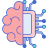# Machine Learning in Linux: scikit-learn – machine learning library for Python

#### In Operation

scikit-learn features classification, regression and clustering algorithms including support-vector machines, random forests, gradient boosting, k-means and DBSCAN.

The project’s website hosts lots of example code. By way of illustration, let’s look at a couple of interesting machine learning examples for the sklearn.gaussian_process module. This module implements Gaussian Process based regression and classification. Gaussian Processes (GP) are a generic supervised learning method designed to solve regression and probabilistic classification problems.

We’ll download an example with wget which illustrates Gaussian Process Classification on XOR data.

`\$ wget https://scikit-learn.org/stable/_downloads/08fc4f471ae40388eb535678346dc9d1/plot_gpc_xor.py`

We run the Python script with the command:

`\$ python plot_gpc_xor.py`

Here’s the output.

The next example also uses the sklearn.gaussian_process module. This example illustrates the predicted probability of GPC for an isotropic and anisotropic RBF kernel on a two-dimensional version for the iris-dataset.

`\$ wget https://scikit-learn.org/stable/_downloads/44d6b1038c2225e954af6a4f193c2a94/plot_gpc_iris.py`

`\$ python plot_gpc_iris.py`

#### Summary

scikit-learn is one of the most commonly used packages when it comes to Machine Learning and Python. The library is simple to use and efficient as it is built on NumPy, SciPy and matplotlib.

It allows us to define machine learning algorithms and compare them to one another, as well as offers tools to preprocess data. It comes with a few standard datasets, for instance the iris and digits datasets for classification and the diabetes dataset for regression.

The software includes models for K-means clustering, Random Forests, Support Vector Machines, and any other machine learning model we want to develop with its tools.

Before you start using scikit-learn you’ll need some experience with Python’s syntax, Pandas, NumPy, SciPy and data analysis in Python. You’ll also need some experience of selecting algorithms, parameters, and sets of data to optimize the results of the method.

Website: scikit-learn.org
Support: GitHub Code Repository
Developer: Team of volunteers

scikit-learn is written in Python. Learn Python with our recommended free books and free tutorials.For other useful open source apps that use machine learning/deep learning, we’ve compiled this roundup.